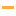# Math Games

Play the best free online Math Games! Check out the Top 100 Math games from PrimaryGames.

#61Math Car
#62MathcopterTry to fly through all five levels while avoiding the parachutes with the wrong answers. Collect smiley faces for bonus points and avoid other objects.

#63Mathcopter 2Try to fly through all five levels while flying into balloons containing the fractions that are equal to the fraction at the bottom of the screen.

#64Math Eggs: Factoring NumbersCatch eggs from chickens that are factors of the numbered label on the basket. Your score will be decreased if you catch numbers that are not factors. You have 3 lives at the start of the game. Lose a life when an egg from a chicken showing a correct fact

#65Math Eggs: Prime NumbersCatch eggs from chickens that are prime numbers. Your score will be decreased if you catch composite numbers (not prime). You have 3 lives at the start of the game. Lose a life when an egg from a chicken showing a prime number breaks on the ground.

#66MathepillarMathepillar is an outrageously fun game in which players have to order falling numbers on a number line by positioning their caterpillar in the correct place to catch the numbers. There are four stages to the game, and each stage presents a slightly more

#67Math HurdlesAnswer simple math questions to jump hurdles. A fun math training game for grade 1 through 3.

#68Math LeaperLeap and jump into power ups and candies to unlock new heights and achievements by answering math questions correctly. Test your math skills while avoiding nasty slime that will ruin your high flying adventure.

#69Math Man
#70Math Maze: CountingPractice counting in the Math Maze. Start in the upper left corner and move through the maze by clicking on the number that comes next.

#71Math Memory Clocks: Christmas EditionMatch the analog clocks with the correct time to make a Christmas picture.

#72Math Memory Clocks: Easter EditionMatch the analog clocks with the correct time to make an Easter picture.

#73Math Memory Clocks: Spring EditionMatch the analog clocks with the correct time to make a Spring picture.

#74Math Memory Clocks: Digital Clocks
#75Math Mosaics: AutumnIt's color by number! To determine the color of each space solve each addition problem, then use the code at the side of the page. The final picture will be revealed as each square is filled.

#76Math Mosaics: ChristmasIt's color by number! To determine the color of each space solve each addition problem, then use the code at the side of the page. The final picture will be revealed as each square is filled.

#77Math Mosaics: HalloweenIt's color by number! To determine the color of each space solve each addition problem, then use the code at the side of the page. The final picture will be revealed as each square is filled.

#78Math Mosaics: SpringIt's color by number! To determine the color of each space solve each addition problem, then use the code at the side of the page. The final picture will be revealed as each square is filled.

#79Math Mosaics: Valentine's DayIt's color by number! To determine the color of each space solve each addition problem, then use the code at the side of the page. The final picture will be revealed as each square is filled.

#80Math Mosaics: WinterIt's color by number! To determine the color of each space solve each addition problem, then use the code at the side of the page. The final picture will be revealed as each square is filled.